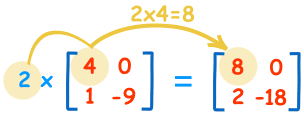# Scalar Multiplication of Matrix

Let A be any matrix. Let k be any non-zero scalar. The matrix kA is obtained by multiplying all the entries of matrix A by the non-zero scalar k.

A = [aij]m×n

⇒ kA = [kaij]m×n

This is called scalar multiplication of a matrix.

If a matrix A is of order m×n, then the matrix kA is also of the same order m×n.